How to Calculate Deciles in Excel?

• Last Updated : 30 Nov, 2021

Decile is a statistical method to split data into 10 equal parts.  Each represents 1/10 of the Population or Sample data. It is denoted D1, D2, … D9.  Fifth deciles D5 are equal to the median.  It determines 10%,20%, … 90%.

Deciles Formula: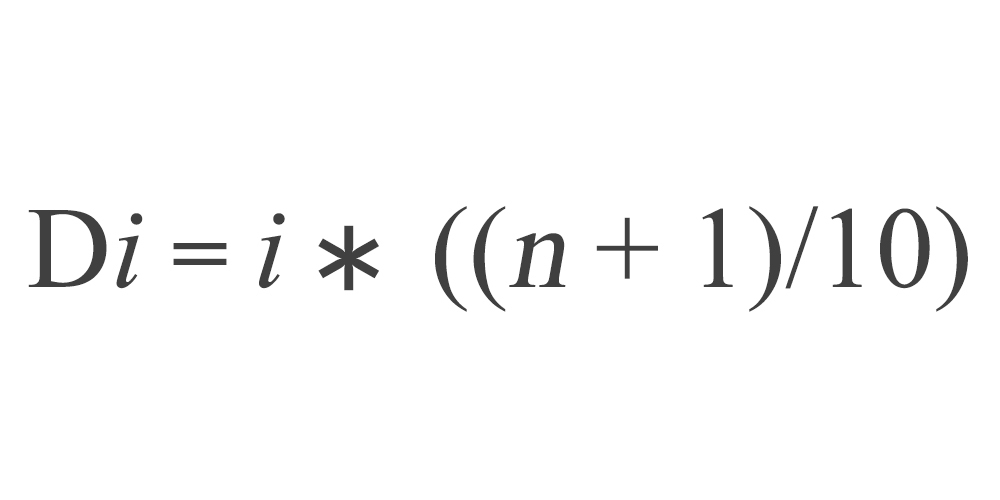Here,

• n: Number of data points in the population or sample (In the below eg. n = 25)

Find the position of Deciles

D1 = 1*((25+1)/10) = 1*(26/10) = 1*(2.6) = 2.6th value [data between 2 and 3 ]
D2 = 2*((25+1)/10) = 2*(26/10) = 2*(2.6) = 5.2nd value [data between 5 and 6 ]

Sample Data: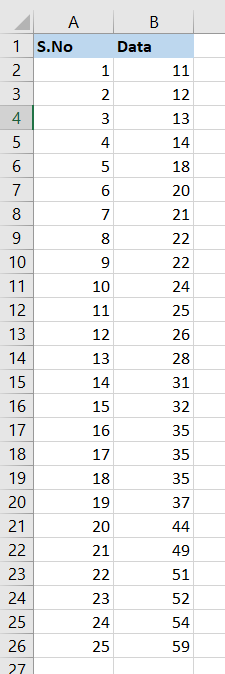Implementation:

Follow the below steps to calculate the Deciles in Excel:

Step 1: Sort the data in ascending order.

Step 2: Compute n (Number of data points); in the above example n is 25, which is placed in cell “F1”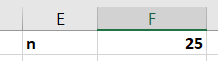Step 3: Write Headers for decile table as below in cells E3, F3 and G3.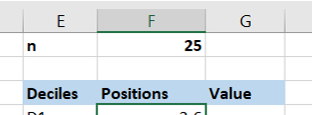Step 4: Type text D1 to D9 in cells “E4 to E12” respectively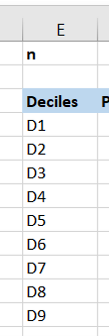Step 5: In cells “F4 to F12” fill decile formula for the respective deciles to find the positions

D1 =1*(\$F\$1+1)/10
D2 =2*(\$F\$1+1)/10
D3 =3*(\$F\$1+1)/10
D4 =4*(\$F\$1+1)/10
D5 =5*(\$F\$1+1)/10
D6 =6*(\$F\$1+1)/10
D7 =7*(\$F\$1+1)/10
D8 =8*(\$F\$1+1)/10
D9 =9*(\$F\$1+1)/10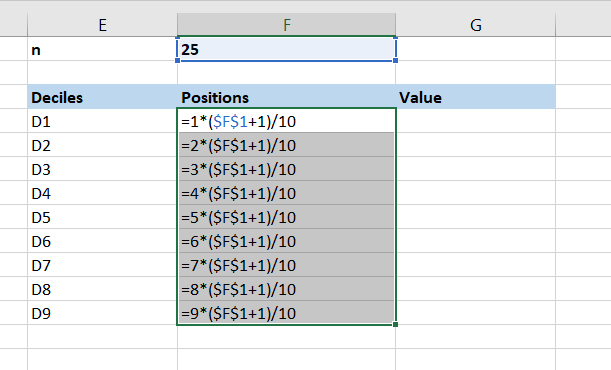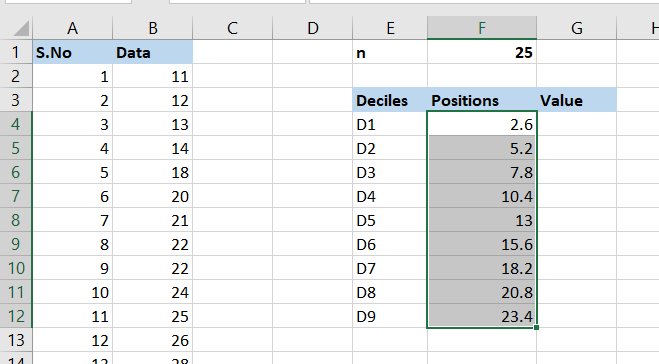Step 6: Decile value calculation for the respective positions

For D1 2.6th Position
find 2nd observation – 12
find 3rd observation – 13
2nd observation + (3rd observation-2nd observation) * 0.6
12 + (13-12) *0.6 = 12.6

Write below formula in cells G4 to G12 for D1 to D9

=B3+(B4-B3)*0.6
=B6+(B7-B6)*0.2
=B7+(B8-B7)*0.8
=B11+(B12-B11)*0.4
=B14+(B14-B14)*0
=B16+(B17-B16)*0.6
=B19+(B20-B19)*0.2
=B21+(B22-B21)*0.8
=B24+(B25-B24)*0.4

Output: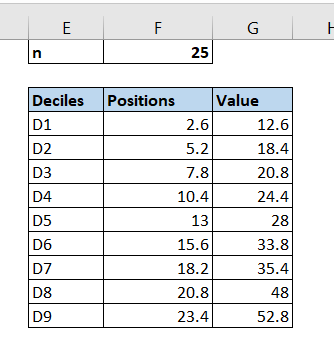My Personal Notes arrow_drop_up